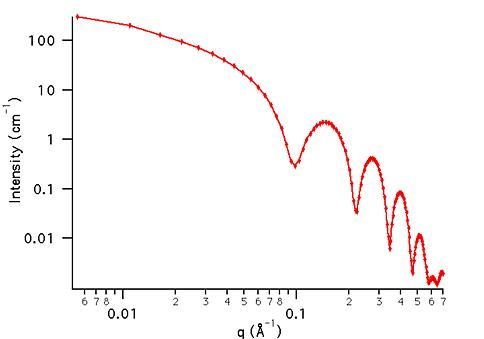MODEL

CoreShellCylinder

ORIGINAL AUTHOR/MODIFICATION

Steve Kline 20 JAN 1999

Alan Munter 08 JULY 1999, converted to Java

APPROVED FOR DISTRIBUTION

DESCRIPTION

Calculates the form factor for a monodisperse, right circular cylinder with a core-shell scattering length density profile. The shell thickness, t, is considered to be uniform over the entire surface of the core. The form factor is normalized by the total particle volume such that P(q) = scale*<f*f>/Vol + bkg, where f is the scattering amplitude and the < > denote an average over all possible orientations of the cylinder. Resolution smeared version is also provided.

VARIABLES

Input Variables (default values):

Parameter Variable Value
0Scale1.0
2Shell Thickness (Å)10.0
3Core Length (Å)400.0
4Core SLD (Å-2)1.0e-6
5Shell SLD (Å-2)4.0e-6
6Solvent SLD (Å-2)1.0e-6
7incoherent Background(cm-1)0.000

USAGE NOTES

The function calculated is: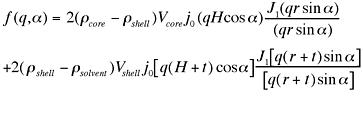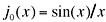,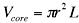and;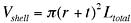where r is the radius of the core of the cylinder as shown below, and J1(x) is the first order bessel function. Alpha is defined as the angle between the cylinder axis and the scattering vector, q. The integral over alpha averages the form factor over all possible orientations of the cylinder. The returned value is scaled to units of [cm-1], on absolute scale.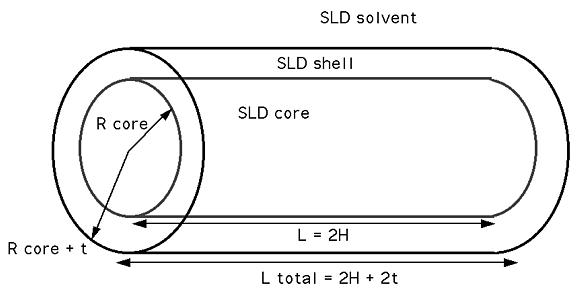The shell thickness is uniform over the entire particle. This means that for the default case above, the total diameter of the cylinder is 2*20 + 2*10 = 60 Å and that the total length is 400 + 2*10 = 420 Å. The calculated cylinder volume is based on these total cylinder dimensions.

The constrasts in the default case correspond to scattering from a cylindrical shell.

Parameter (scale) and Parameter, Parameter, and Parameter (SLD's) are multiplicative factors in the model and are perfectly correlated. Only one of these four parameters should be left free during model fitting.

If the scale factor, Parameter, is set equal to the particle volume fraction, phi, the the returned value is the scattered intensity per unit volume, I(q) = phi*P(q). However, no interparticle interference effects are included in this calculation.

REFERENCE

TEST DATASET

This example dataset is produced by calculating the CoreShellCylinder, using 128 data points, qmin = 0.001 Å-1, qmax = 0.7 Å-1 and the above default coefficient values.